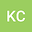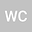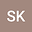Saturation-ratio Fluctuations from Scalar Transport in Moist Rayleigh-Bénard Convection: One-dimensional-turbulence simulation
•••• +2Scott Wunsch
Johns Hopkins University
Author Profile## Abstract

A careful characterization of moisture fluxes and saturation-ratio statistics in atmospheric convection is significant for cloud microphysical processes and dynamics. The saturation-ratio of water vapor is defined as the ratio of actual water vapor pressure and its equilibrium value at a given air temperature. Therefore, it is a function of two scalars (water vapor and temperature) and is coupled through the nonlinear Clausius-Clapeyron equation. Participation of both scalar fields in the convection process and the nonlinear coupling of both scalars in saturation-ratio make this problem more complex, as compared to its dry-convection counterpart. We have explored heat and water vapor fluxes and saturation-ratio statistic in the moist Rayleigh-Bénard convection case, using the one-dimensional-turbulence (ODT) model developed by Wunsch et al. JFM 2005. This idealized small-scale simulation is a step toward understanding the full atmospheric convection problem at a more fundamental level. We have obtained the thermal and moisture fluxes as a function of the non-dimensional buoyancy parameter, also known as moist Rayleigh number, and compared it with the scaling relations. Moreover, we have examined the mean and variance profiles of saturation-ratio, and analyzed the different contributing terms for saturation-ratio fluctuations. Based on the scaling analysis, a simplified relation between saturation-ratio variance and moist Rayleigh number has been derived and compared with the simulation results. Additionally, we found that different values of water vapor and thermal diffusivities make the saturation-ratio pdf broader than the case when they are considered equal.